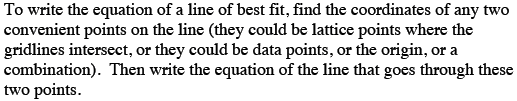Home > CCA > Chapter 4 > Lesson 4.1.1 > Problem4-8

4-8.

Smallville High School Principal is concerned about his school’s Advanced Placement (AP) test scores. He wonders if there is a relationship between the students’ performance in class and their AP test scores so he randomly selects a sample of ten students who took AP examinations and compares their final exam scores to their AP test scores. 4-8 HW eTool (Desmos). Homework Help ✎

 AP Score $5$ $1$ $4$ $2$ $1$ $4$ $2$ $1$ $3$ $5$ Final Score $\%$ $97$ $70$ $84$ $66$ $62$ $79$ $73$ $63$ $82$ $90$

Create a scatterplot on graph paper. Draw a line of best fit that represents the data. Refer to the Math Notes box in this lesson. Use the equation of your line of best fit to predict the final exam score of another Smallville HS student who scored a $3$ on their AP test.

A line of best fit is a straight line that represents data on a scatter plot. It does not need to touch any of the data points, nor does it need to go through the origin.

Refer to this section of the math notes: Answers may vary.Complete the table in the eTool below to graph the results.
Click the link at right for the full version of the eTool: CCA 4-8 HW eTool